Author: Oscar Cronquist Article last updated on May 11, 2023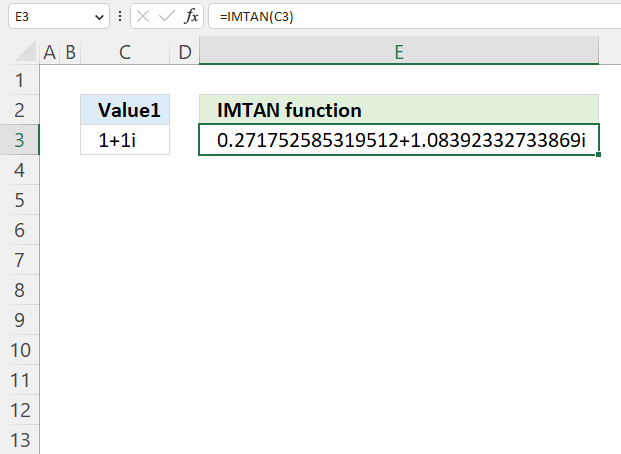What is the IMTAN function?

The IMTAN function calculates the tangent of a complex number in x + yi or x + yj text format.

The letter j is used in electrical engineering to distinguish between the imaginary value and the electric current.

IMTAN(inumber)

## 2. IMTAN Function Arguments

 inumber Required. A complex number in x+yi or x+yj text format.

## 3. IMTAN function example 1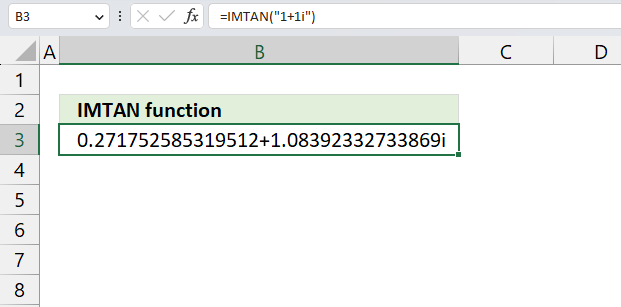The image above demonstrates a formula in cell D3 that calculates the tangent of a complex number in general or rectangular form specified in the function.

=IMTAN("1+1i")

The IMTAN probably stands for imaginary tangent.

### 3.1 What are complex numbers?

Complex numbers allow us to solve equations that have no real solutions. Complex number have two parts, a real part and an imaginary part called i which means imaginary and stands for the square root of -1.

### 3.2 What are the applications for complex numbers?

Complex numbers are very useful in many fields of mathematics and science.

• Electronics: Complex numbers are used to analyze circuits that involve alternating current (AC) or signals modulated by electromagnetic waves.
• Electromagnetism: Complex numbers are used to describe the electric and magnetic fields in terms of their magnitude and direction.
• Control theory: Complex numbers are used to design and optimize systems that have feedback loops, such as robots, airplanes, or rockets.
• Signal analysis: Complex numbers are used to perform Fourier transforms, which are methods of decomposing a signal into its frequency components.
• Relativity: Complex numbers are used to express the space-time interval in special relativity, which is a measure of the distance between two events that occur in different locations and times.
• Fluid dynamics: Complex numbers are used to model the flow of fluids, such as air or water.

## 4. How is the IMTAN function result calculated?

z - complex number: 1+1i
a - real number: 1
b - imaginary number: 1

The math formula to calculate the imaginary tangent is: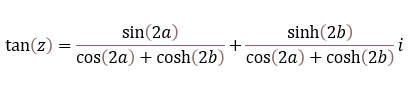#### Step 1 - Calculate real sin

The SIN function calculates the sine of an angle.

Function syntax: SIN(number)

sin(2a)

becomes

sin(2*1)

and returns 0.909297426825682

#### Step 2 - Calculate real cos

The COS function calculates the cosine of an angle.

Function syntax: COS(number)

cos(2a)

becomes

cos(2*1)

and returns -0.416146836547142

#### Step 3 - Calculate imaginary sinh

The SINH function calculates the hyperbolic sine of a number.

Function syntax: SINH(number)

sinh(2b)

becomes

sinh(2*1)

and returns 3.62686040784702

#### Step 4 - Calculate imaginary cosh

The COSH function calculates the hyperbolic cosine of a number.

Function syntax: COSH(number)

cosh(2b)

becomes

cosh(2*1)

and returns 3.76219569108363

#### Step 5 - Divide first quotient

0.909297426825682/(-0.416146836547142+3.76219569108363)

becomes

0.909297426825682/3.34604885453649

and returns 0.271752585319512

#### Step 6 - Divide second quotient

3.62686040784702/(-0.416146836547142+3.76219569108363)

becomes

3.62686040784702/3.34604885453649

and returns 1.08392332733869iThe calculations match the result from the IMTAN function.

## 5. IMTAN function example 2 - cell referenceThe image above demonstrates a formula in cell D3 that calculates the tangent of a complex number specified in cell C3.

Formula in cell D3:

=IMTAN(C3)

### 5.1 Explaining formula

IMTAN(inumber1)

becomes

IMTAN(C3)

#### Step 2 - Evaluate the IMTAN function

IMTAN(C3)

becomes

IMTAN("1+1i")

and returns

0.271752585319512+1.08392332733869i

## 6. IMTAN function example 3 - real and imaginary coefficients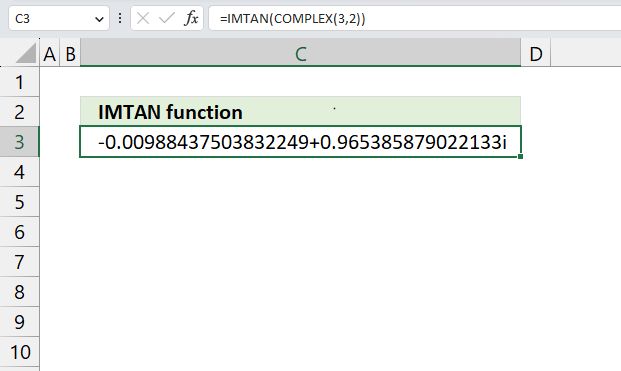The image above demonstrates a formula in cell D3 that calculates the tangent of real and imaginary coefficients specified in the COMPLEX function. The COMPLEX function lets you create a complex number using a real and imaginary number.

Formula in cell D3:

=IMTAN(COMPLEX(3,2))

### 6.1 Explaining formula

#### Step 1 - Calculate complex number based on a real and imaginary number

The COMPLEX function returns a complex number based on a real and imaginary number.

Function syntax: COMPLEX(real_num, i_num, [suffix])

COMPLEX(3,2)

returns

"3+2i".

#### Step 2 - Calculate the tangent of a complex number

IMTAN(COMPLEX(3,2))

becomes

IMTAN("3+2i")

and returns

-0.009+ 0.965i

## 7. IMTAN function error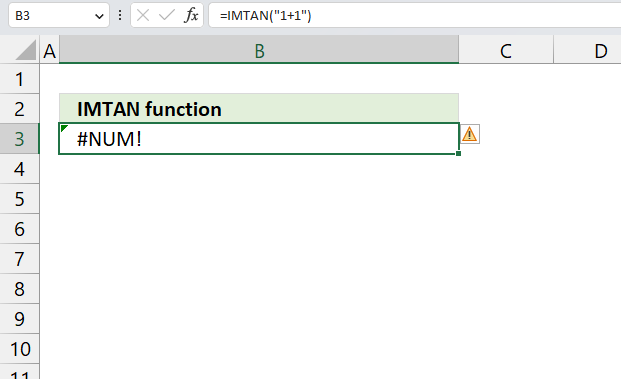The IMTAN function returns a #NUM error if we use an invalid argument. Make sure the argument is valid.

The IMTAN function returns #NUM error if the suffix is not "i" or "j", or omitted.